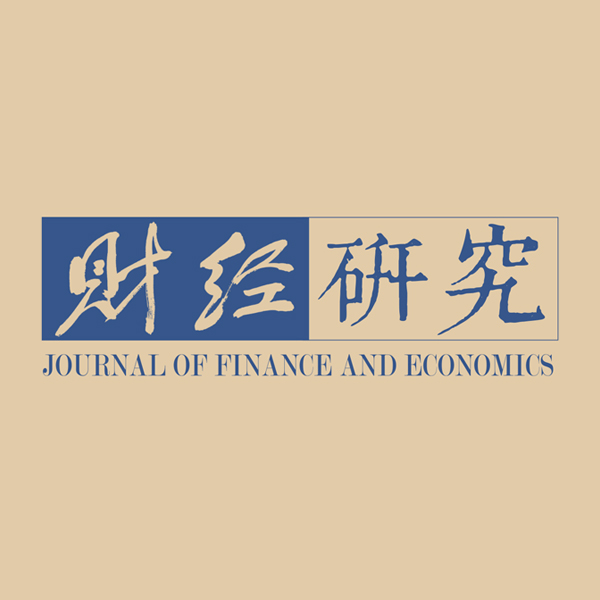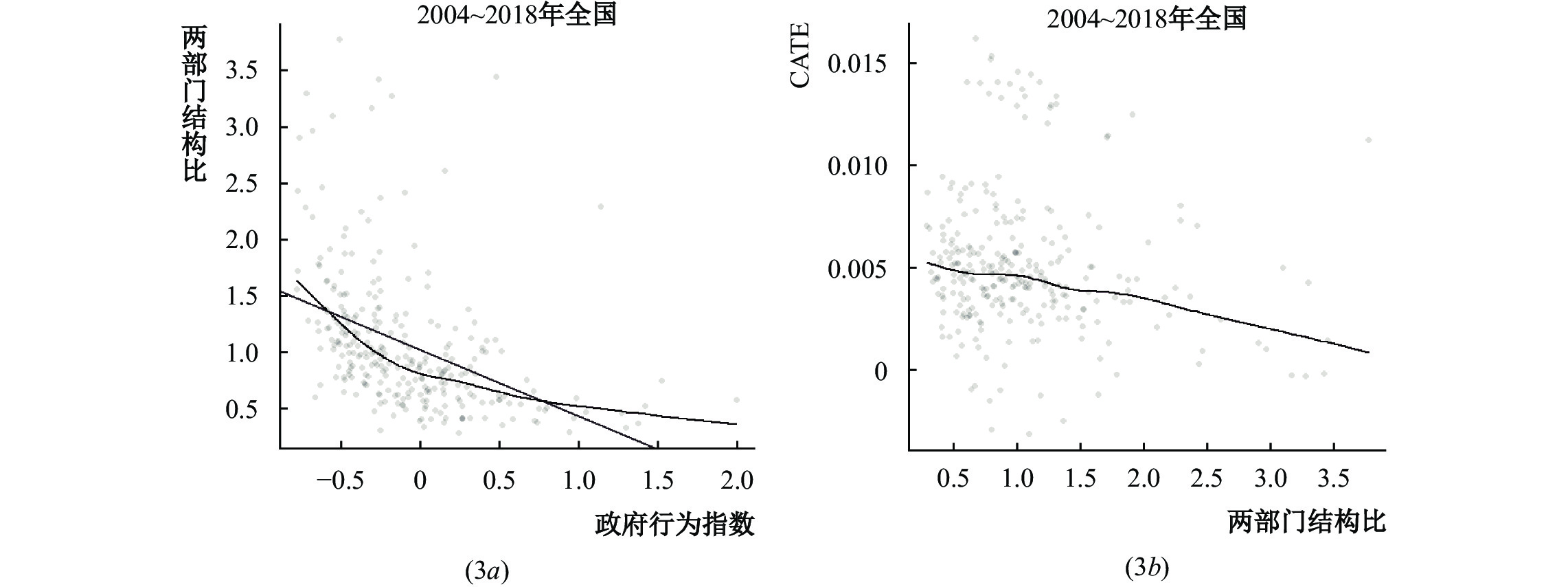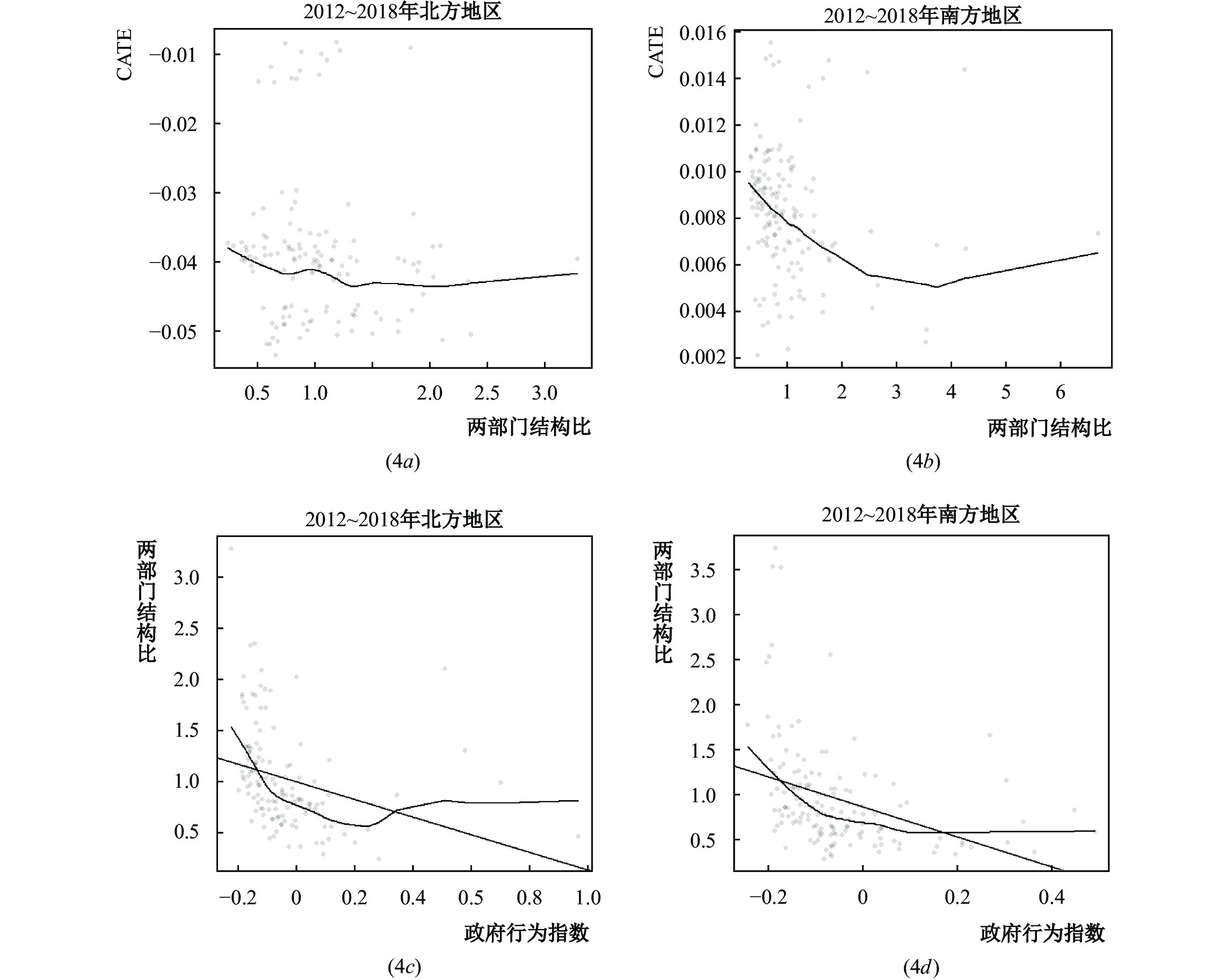﻿ “倾斜性”政策、生产部门变迁与南北地区发展差异
>《财经研究》
2022第48卷第1期
“倾斜性”政策、生产部门变迁与南北地区发展差异——来自机器学习的因果推断

1. 长沙理工大学 经管学院, 湖南 长沙 410114;
2. 湖南大学 经贸学院, 湖南 长沙 410006

“Biased” Policy, Changes of Production Sectors and Development Gap between the North and the South in China: Causal Inference from Machine Learning
Hu Zunguo1, Gu Jinxin1, Chen Ying2
1. School of Economics and Management, Changsha University of Science and Technology, Changsha 410114, China;
2. School of Economics and Trade, Hunan University, Changsha 410006, China
Summary: Over the past decade, China’s regional policies have significantly narrowed the per capita output gap between inland and coastal areas, but the latest development differentiation between the North and the South has attracted much attention from different fields. Starting from judging the heterogeneous effect of “biased” policy, this paper deeply analyzes the impact of “biased” policy on the structural change of production sectors, and then explains the differences found in the North and the South, which further enriches the economic growth and China’s regional development theory under the parallel background of “effective market” and “active government”. The “biased” policy covers non-productive regional policies, as well as productive regional policies based on balanced development, such as project priority. With the help of “biased” policy, many regions have the trend of the rapid expansion of industrial scale and accelerated urbanization in the short term, but more active involvement in economic activities is easy to lead to the expansion of the share of non-tradable production sectors. Subject to the low degree of the marketization of non-tradable sectors, the disadvantage of relatively slow productivity improvement in regions with expanding share of the sector will be exposed. In other words, the “biased” policy can have an asymmetric shock on the structure of local production sectors, and the behavior of local governments often aggravates the spatial heterogeneity of this shock, which forms another new source of regional development differentiation in China. The results show that: (1) The effect of policies aimed at balanced development in the South is better than that in the North, especially after 2012. (2) The “biased” policy will increase the share of non-tradable sectors in underdeveloped areas, resulting in a relatively slow increase in long-term productivity compared with tradable sectors. Although this phenomenon is common in China, it is relatively more serious in the North, which is an important reason for the widening development gap between the North and the South. This paper has the following contributions: (1) It firstly discusses urban productivity through the channel between “biased” policy and production sector structure, and then explores the reasons for regional development gap. (2) It constructs an equilibrium model of “biased” policy, two sector structure and regional development for the first time. (3) Using machine learning to carry out empirical analysis on the high-dimensional characteristics of 277 cities from 2003 to 2018, the heterogeneity treatment effect of “biased” policy is estimated by causal forest for the first time.
Key words: machine learning    causal forest    regional policies    production sector structure

$u = \lambda {}_k\ln {c_k} + \lambda {}_u\ln {c_u}$ (1)

${\delta _j} = \frac{{{L_{Mj}}}}{{{L_{Nj}}}} \text{，} j = k,u$ (2)

${L_{Mj}}$ 为发达地区 $M$ 生产部门 $j$ 劳动人口占该部门全国劳动人口份额， ${L_{Nj}}$ 为欠发达地区 $N$ 生产部门 $j$ 劳动人口占该部门全国劳动人口份额， ${L_{Mj}} + {L_{Nj}} = 1$ 。而各部门的生产效率在地区之间有所区别，两地区可贸易部门生产效率相对比值 ${\tau _k}$ 与不可贸易部门生产效率相对比值 ${\tau _u}$ 表示为:

 ${\tau _j} = \frac{{{A_{Mj}}}}{{{A_{uj}}}} \text{，} j = k,u$ (3)

${\zeta _i} = \frac{{{L_{ik}}}}{{{L_{iu}}}} \text{，} i = M,N$ (4)

${L_{ik}}$ ${L_{iu}}$ 分别为地区 $i$ 可贸易部门和不可贸易部门的劳动人口在该地区总就业人口里所占的份额， ${L_{ik}} + {L_{iu}} = 1$ 。同一地区不同部门生产率也存在差别，发达地区两部门生产率相对比值 ${\psi _M}$ 和欠发达地区两部门生产率相对比值 ${\psi _N}$ 表示如下：

${\psi _i} = \frac{{{A_{ik}}}}{{{A_{iu}}}} \text{，} i = M,N$ (5)

$i$ 地区可贸易部门产出函数表示为：

${Y_{ik}} = {A_{ik}}L_{ik}^{1 - \alpha } \text{，} i = M,N$ (6)

${Y}_{k}={Y}_{Mk}+{Y}_{Nk}={A}_{Mk}{L}_{Mk}^{1-\alpha }+{A}_{Nk}{L}_{Nk}^{1-\alpha }=（{\tau }_{k}+{\delta }_{k}{}^{1\text-\alpha }）{\psi }_{N}{\zeta }_{N}^{1-\alpha }{A}_{Nu}{L}_{Nu}^{1-a}$ (7)

$i$ 地区不可贸易部门产出函数表示为：

${Y_{iu}} = {A_{iu}}L_{iu}^{1 - \alpha } \text{，} i = M,N$ (8)

${Y}_{u}={Y}_{Mu}+{Y}_{Nu}={A}_{Mu}{L}_{Mu}^{1-\alpha }+{A}_{Nu}{L}_{Nu}^{1-\alpha }=（{\tau }_{u}+{\delta }_{u}^{1\text-\alpha }）{A}_{Nu}{L}_{Nu}^{1-a}$ (9)

${Y_M} = {Y_{Mk}} + {Y_{Mu}} = {A_{Mu}}L_{Mu}^{1 - \alpha } + {A_{Mk}}L_{Mk}^{1 - \alpha } = {\tau _u}\delta _u^{1 - \alpha }({\psi _M}\zeta _M^{1 - \alpha } + 1){A_{Nu}}L_{Nu}^{1 - \alpha }$ (10)

${Y_N} = {Y_{Nk}} + {Y_{Nu}} = {A_{Nu}}L_{Nu}^{1 - \alpha } + {A_{Nk}}L_{Nk}^{1 - \alpha } = (1 + {\psi _N}\zeta _N^{1 - \alpha }){A_{Nu}}L_{Nu}^{1 - \alpha }$ (11)

$p_{ik}^{*}{c_{ik}} + {p_{iu}}{c_{iu}} = {q_i} \text{，} i = M,N$ (12)

 ${\lambda _{iu}}{q_i} = {\theta _i}{R_i} + {p_{iu}}{Y_{iu}} \quad i = M,N$ (13)
 ${\lambda _{ik}}{q_i} = (1 - {\theta _i}){R_i} + p_{ik}^*{Y_{ik}} \text{，} i = M,N$ (14)

 ${w_{Mj}} = {w_{Nj}} , j = k,u$ (15)

 ${w_{ik}} = {w_{iu}} \text{，} i = M,N$ (16)

${L_{iu}} = {\left\{ \frac{{[(1 - {\theta _i}){\lambda _{iu}} + {\theta _i}{\lambda _{ik}}] \cdot {R_i}}}{{({\lambda _{ik}} - {\lambda _{iu}}{\zeta _i}){P_{iu}}{A_{iu}}}}\right\} ^{ {{\raise0.3ex\hbox{$1$} \mathord{\left/ {\vphantom {1 {1 - \alpha }}}\right.\kern-\nulldelimiterspace} \lower0.3ex\hbox{${1 - \alpha }$}}}}} , i = M,N$ (17)
${Y_{iu}} = \frac{{[(1 - {\theta _i}){\lambda _{iu}} + {\theta _i}{\lambda _{ik}}] \cdot {R_i}}}{{({\lambda _{ik}} - {\lambda _{iu}}{\zeta _i}){P_{iu}}}} , i = M,N$ (18)
${L_{ik}} = {\left\{ \frac{{[(1 - {\theta _i}){\lambda _{iu}} - {\theta _i}{\lambda _{ik}}] \cdot {\zeta _i}{R_i}}}{{({\lambda _{ik}} - {\lambda _{iu}}{\zeta _i}){\psi _i}P_k^*{A_{iu}}}}\right\} ^{ {{\raise0.7ex\hbox{$1$} \mathord{\left/ {\vphantom {1 {1 - \alpha }}}\right.\kern-\nulldelimiterspace} \lower0.7ex\hbox{${1 - \alpha }$}}}}} , i = M,N$ (19)
${Y_{ik}} = \frac{{[(1 - {\theta _i}){\lambda _{iu}} - {\theta _i}{\lambda _{ik}}] \cdot {\zeta _i}{R_i}}}{{({\lambda _{ik}} - {\lambda _{iu}}{\zeta _i}){\psi _i}P_k^*}} , i = M,N$ (20)

$（1+{\tau }_{k}{\delta }_{k}^{1-\alpha }）{P}_{k}^{*}{A}_{Nk}{L}_{Nk}^{-\alpha }=（1+{\tau }_{u}{\delta }_{u}^{1-\alpha }）{P}_{u}{A}_{Nu}{L}_{Nu}^{-\alpha }$ (21)

$\gamma = \frac{{{Y_M}}}{{{Y_N}}} = \frac{{{Y_{Mk}} + {Y_{Mu}}}}{{{Y_{Nk}} + {Y_{Nu}}}} = \frac{{{\tau _u}\delta _u^{1 - \alpha }({\psi _M}\zeta _M^{1 - \alpha } + 1)}}{{{\psi _N}\zeta _N^{1 - \alpha } + 1}}$ (22)

$\gamma = \frac{{{{\left[ {{\lambda _u}{R_N}{P_{Nu}}({\theta _N}{A_{Nu}} - {A_{Nk}})} \right]}^{1 - \alpha }} + {\theta _M}{{({\psi _M}\zeta _M^{ - \alpha } + {R_M}{\lambda _k}P_k^{*})}^{1 - \alpha }}}}{{{\psi _N}\zeta _N^{1 - \alpha } + {\delta _u}(\tau _k/{\tau _u})}}$ (23)

${\lambda _{iu}} = {\lambda _u},{\lambda _{ik}} = {\lambda _k}$ (24)

${L_{iu}}$ ${L_{ik}}$ $\gamma$ 分别关于 $R$ $\theta$ 偏导，可以得到：

$\frac{{\partial {L_{iu}}}}{{\partial {R_i}}} = {(1 - \alpha )^{ - 1}}{R_i}^{\alpha/1-\alpha}{\left[\frac{{(1 - {\theta _i}){\lambda _{iu}} + {\theta _i}{\lambda _{ik}}}}{{({\lambda _{ik}} - {\lambda _{iu}}{\zeta _i}){P_{iu}}{A_{iu}}}}\right]^{1/1-\alpha}}$ (25)
$\frac{\partial {L}_{iu}}{\partial {\theta }_{i}}={(1-\alpha )}^{-1}\left(\frac{{R}_{i}}{{P}_{iu}{A}_{iu}}\right)^{1/1-\alpha}\left(\frac{{\lambda }_{ik}-{\lambda }_{iu}}{{\lambda }_{ik}-{\lambda }_{iu}{\zeta }_{i}}\right){\left[\frac{(1-{\theta }_{i}){\lambda }_{iu}+{\theta }_{i}{\lambda }_{ik}}{({\lambda }_{ik}-{\lambda }_{iu}{\zeta }_{i})}\right]}^{\alpha/1-\alpha}$ (26)
$\frac{{\partial {L_{ik}}}}{{\partial {R_i}}} = {(1 - \alpha )^{ - 1}}{R_i}^{\alpha/1}{\left[\frac{{[(1 - {\theta _i}){\lambda _{iu}} - {\theta _i}{\lambda _{ik}}]{\zeta _i}}}{{({\lambda _{ik}} - {\lambda _{iu}}{\zeta _i}){\psi _i}P_k^*{A_{iu}}}}\right]^{1/1-\alpha}}$ (27)
$\frac{\partial {L}_{ik}}{\partial {\theta }_{i}}={(1-\alpha )}^{-1}\left(\frac{{\zeta }_{i}{R}_{i}}{{\psi }_{i}{P}_{k}^{*}{A}_{iu}}\right)^{1/1-\alpha}\left(\frac{-{\lambda }_{ik}-{\lambda }_{iu}}{{\lambda }_{ik}-{\lambda }_{iu}{\zeta }_{i}}\right){\left[\frac{(1-{\theta }_{i}){\lambda }_{iu}-{\theta }_{i}{\lambda }_{ik}}{({\lambda }_{ik}-{\lambda }_{iu}{\zeta }_{i}){\psi }_{i}}\right]}^{\alpha/1-\alpha}$ (28)
$\frac{{\partial \gamma }}{{\partial {R_N}}} = \frac{{(1 - \alpha ){R_N}^{ - \alpha }{{\left[ {{\lambda _u}{P_{Nu}}({\theta _N}{A_{Nu}} - {A_{Nk}})} \right]}^{1 - \alpha }}}}{{{\psi _N}\zeta _N^{1 - \alpha } + {\delta _u}(\tau _k/{\tau _u})}}$ (29)

（i） $\dfrac{{\partial {L_{Nu}}}}{{\partial {R_N}}} > 0$ $\dfrac{{\partial {L_{Nu}}}}{{\partial {\theta _N}}} > 0$ ，而 $\dfrac{{\partial {L_{Nk}}}}{{\partial {R_N}}}$ $\dfrac{{\partial {L_{Nk}}}}{{\partial {\theta _N}}}$ 符号是不确定的。

（ii）当 ${\theta _N} > \dfrac{{{A_{Nk}}}}{{{A_{Nu}}}}$ ，即 ${\theta _N}{A_{Nu}} - {A_{Nk}} > 0$ 时， $\dfrac{{\partial \gamma }}{{\partial {R_N}}} > 0$ ；当 ${\theta _N} < \dfrac{{{A_{Nk}}}}{{{A_{Nu}}}}$ ，即 ${\theta _N}{A_{Nu}} - {A_{Nk}} < 0$ ，有 $\dfrac{{\partial \gamma }}{{\partial {R_N}}} < 0$ ，两地区发展差距 $\gamma$ 与区域政策租存在非线性关系，该非线性关系取决于地方政府将政策租分配到不可贸易部门的比例为 ${\theta _N}$ 和各部门的生产率（ ${A}_{k}或{A}_{u}$ ）。因此，本文提出待检验的假说如下：

${f_{ij}} = {\sigma _{ij}}\frac{{\sqrt {{q_i}{q_j}} }}{{{d_{ij}}^2}} \text{，} {\sigma _{ij}} = \frac{{{q_j}}}{{{q_i} + {q_j}}}$ (30)

 变量名称 具体指标 依据或构建说明 地理区位 南北区位，以秦岭—淮河为界划分南北方城市① 分类数据，北方城市设为0，南方城市设为1 板块区域，按照年鉴划分四大区域 分类数据，东部地区城市设为4，东北城市设为3，中部地区城市设为2，西部地区城市设为1 港口距离，这里基于可利用港口的便利性来衡量 定序数据，港口城市设为4，沿海省份的其他非港口城市设为3，中部和东北地区城市设为2，其他城市设为1 经济发展禀赋 2003年人均GDP 经济初始基本面，也是区域协调政策的参考依据 金融状况 信贷增速 用贷款规模增长率表示 人力资本 人力资本 城市普通高校在校人数/城市年末总人口 运输条件 货运量 用公路货运量衡量，该指标一定程度上反映了城市的运输能力 投资规模 固定资产投资 全社会固定资产投资总额 政府行为指数 建设用地面积增速 反映了政府对要素流动、企业选择和产业扩张产生的影响 建设用地面积变异系数 用一定时间段内城市建设用地面积变异系数衡量，例如2004—2011年某城市建设用地面积数量的标准差除以其平均值，若这种相对波动较强，意味政府干预力度较大 财政收支比 用财政支出/财政收入表示 城市规模 辖区人口 用市辖区人口来衡量，城市规模是影响城市经济产出的重要因素 两部门 结构 可贸易部门与不可贸易部门②之比 可贸易就业人数/不可贸易就业人数 城市引力流 2004—2011年引力流 有向关系数据，可用于城市网络分析 2012—2018年引力流

（一）因果森林算法估计过程图 1 地区初始经济禀赋与倾向值的关系

$\hat{\tau }=\frac{{\sum }_{i=1}^{n}{\alpha }_{i}(x)\left[{Y}_{i}-{\hat{m}}^{(-i)}({X}_{i})\right]\left[{Z}_{i}-{\hat{e}}^{(-i)}({X}_{i})\right]}}{{\sum }_{i=1}^{n}{\alpha }_{i}(x){\left[{Z}_{i}-{\hat{e}}^{(-i)}({X}_{i})\right]}^{2}}}$ (31)

${\hat \tau _j} = \frac{{\text{1}}}{{{n_j}}}\sum\limits_{\left\{ {i:{A_i} = j} \right\}} {{{\hat \Gamma }_i}} ,\hat \tau = \frac{1}{J}\sum\limits_{j = 1}^J {{{\hat \tau }_j}} ,{\hat \sigma ^2} = \frac{1}{{J(J - 1)}}{\sum\limits_{j = 1}^J {\left( {{{\hat \tau }_j} - \hat \tau } \right)} ^2}$ (32)
${\hat{\Gamma }}_{i}={\hat{\tau }}^{（\text-i）}（{X}_{i}）+\frac{{Z}_{i}-{\hat{e}}^{(-i)}({X}_{i})}{{\hat{e}}^{(-i)}({X}_{i})\left(1-{\hat{e}}^{(-i)}({X}_{i})\right)}\left\{{Y}_{i}-{\hat{m}}^{(-i)}({X}_{i})-\left[{Z}_{i}-{\hat{e}}^{(-i)}({X}_{i})\right]{\hat{\tau }}^{(-i)}({X}_{i})\right\}$ (33)

（二）基本估计结果

 北方（A） 南方（A） 北方（B） 南方（B） 全阶段ATE 0.006 +/− 0.026 0.024 +/− 0.022 0.005 +/− 0.023 0.024 +/− 0.021 全阶段ATE（聚类） 0.008 +/− 0.029 0.036 +/− 0.025 0.008 +/− 0.028 0.036 +/− 0.025 第一阶段ATE 0.034 +/− 0.034 0.035 +/− 0.027 0.034 +/− 0.03 0.03 +/− 0.019 第一阶段ATE（聚类） 0.041 +/− 0.042 0.052 +/− 0.035 0.04 +/− 0.042 0.051 +/− 0.035 第二阶段ATE −0.029 +/− 0.04 0.009 +/− 0.016 −0.027 +/− 0.037 0.008 +/− 0.015 第二阶段ATE（聚类） −0.029 +/− 0.035 0.015 +/− 0.019 −0.03 +/− 0.035 0.015 +/− 0.018 注：因果森林由1000棵回归树生成，其中表中列名为南方（A）或北方（A）是按照50%比例随机分配训练样本子集后的ATE，南方（B）或北方（B）是按照80%比例随机分配训练样本子集后的ATE。“ +/−”之前的数字表示ATE，“ +/−”后面数字表示5%显著水平下极限误差。这里的第一阶段是2004—2011年，第二阶段是2012—2018年，全阶段是2004—2018年。

（三）“倾斜性”政策效果的南北异质性图 2 CATE分布

（四）生产部门结构变迁图 3 全国部门结构与CATE图 4 南北地区部门结构与CATE

（1） “倾斜性”政策、地方政府行为与城市不可贸易部门份额存在紧密关系。这体现在以下两方面: 一方面，地方政府介入经济活动的力度越大，可贸易与不可贸易两部门就业结构比越小，即不可贸易部门份额更高，如图（3a所示；另一方面，不可贸易部门份额更高的城市，“倾斜性”政策促进其人均GDP增长的效果越好，如图（3b

（2）有关2012—2018年数据统计显示，一方面，不可贸易部门份额与“倾斜性”政策效果的关系因南北区位产生很大差异，不可贸易部门份额更大的南方城市，CATE更大，如图（4b，但北方地区该相关性不明显，如图（4a。另一方面，尽管地方政府介入经济活动都会提高城市不可贸易部门份额，如图（4c（4d，然而受区域政策倾斜的南方城市不可贸易部门份额与CATE的相关性更强，这意味着2012以来“倾斜性”政策对南方城市人均GDP增速仍有促进作用，而北方城市效果不明显。这进一步解释理论假说1和假说2。

（一） 岭回归

 变量 人均GDP（南方） 人均GDP（北方） 人均GDP（全国） Intercept 9.66689 9.59661 8.20483 8.17091 10.09274 11.02740 非东部地区 −0.03897 −0.02053 −0.01444 四大板块 0.03066 0.03933 −0.03684 −0.03499 0.00852 0.01091 港口距离 0.01444 0.01578 −0.01068 −0.00839 −0.02977 −0.02842 2003年人均GDP 0.00003 0.00003 0.00003 0.00003 0.00004 0.00004 信贷发展速度 −0.13373 −0.12260 1.36060 1.36646 −0.31010 −0.30192 人力资本 0.00039 0.00037 0.00032 0.00032 0.00027 0.00026 交通货运量 0.00000 0.00000 0.00000 0.00000 0.00000 0.00000 固定资产投资 0.00000 0.00000 0.00000 0.00000 0.00000 0.00000 建设用地面积增速 −0.02449 −0.02291 −0.02452 −0.02452 −0.01996 −0.01992 建设用地面积变异系数 0.08916 0.08042 −0.06843 −0.06618 0.02759 0.02799 财政收支比 −0.09670 −0.09607 −0.08159 −0.08194 −0.10751 −0.10739 辖区人口 −0.00016 −0.00016 −0.00010 −0.00010 −0.00032 −0.00032 两部门结构比 0.13462 0.13410 0.19442 0.19412 0.12240 0.12221 交叉验证误差最小化 0.04912 0.04912 0.38479 0.38479 0.06804 0.06804 MSE 0.04820 0.04519 0.37563 0.13731 0.04981 0.04976 注：这里的解释变量和被解释变量数据均取自2004—2011年间平均值，其中虚拟变量 ${Z_0}$ 值的设置规则是非东部地区是1，东部地区为0，下表同。

 变量 人均GDP（南方） 人均GDP（北方） 人均GDP（全国） Intercept 12.16557 12.13420 12.15877 12.03631 12.57448 12.55831 非东部地区 −0.02116 −0.02270 −0.00644 四大板块 0.02966 0.03288 −0.07816 −0.07594 −0.03617 −0.03508 港口距离 0.02253 0.02495 −0.03984 −0.03852 −0.01612 −0.01556 2003年人均GDP 0.00002 0.00001 0.00003 0.00003 0.00003 0.00003 信贷发展速度 −1.47377 −1.47564 −1.10582 −1.01122 −1.64251 −1.63668 人力资本 0.00031 0.00030 0.00011 0.00010 0.00020 0.00020 交通货运量 0.00000 0.00000 −0.00001 −0.00001 0.00000 0.00000 固定资产投资 0.00000 0.00000 0.00000 0.00000 0.00000 0.00000 建设用地面积增速 −0.02423 −0.02491 0.00746 0.00805 0.01693 0.01708 建设用地面积变异系数 −0.00139 −0.00142 −0.00191 −0.00164 −0.00102 −0.00101 财政收支比 −0.09688 −0.09427 −0.13114 −0.13361 −0.13415 −0.13414 辖区人口 −0.00002 −0.00001 −0.00042 −0.00044 −0.00034 −0.00034 两部门结构比 0.05098 0.05285 0.04365 0.03963 0.01855 0.01874 交叉验证误差最小化 0.30125 0.33063 0.05477 0.04991 0.04121 0.04121 MSE 0.05804 0.05933 0.04507 0.04431 0.04005 0.04004

（二）基于社会网络分析法（SNA）的检验

（1）“倾斜性”政策一定程度能改变落后地区发展态势，但是在促进经济快速发展过程中，“倾斜性”政策在南方城市效果总体上优于北方，2012年以来该现象更为明显。对不同阶段的因果森林异质性处理效应分析均得到类似的结果，尤其采用省级聚类的因果森林异质性处理效应的稳健性检验结果显示，2004—2018年，“倾斜性”政策干预对南方地区人均GDP发展速度提升年均贡献了3.6%，而北方地区年均贡献约为0.8%。而且，自从2012年以来，“倾斜性”政策在南北地区的效果差别进一步拉大，考虑省份聚类后，南北地区ATE差额从第一阶段0.011扩大到第二阶段0.044。同时，南方城市CATE分布更为集中，受照顾政策城市的生产效率发展规律更趋同，而北方城市的效果出现比较严重的分化；从平均角度看，“倾斜性”政策对北方城市发展的促进效果已逐渐变弱。

（2）生产部门变迁与“倾斜性”政策效果紧密相关。在因果森林形成过程中，2004—2011年北方城市两部门结构比重要性相对更高，而在2012以后该情况发生了逆转。岭回归的结果也显示，两部门结构比是解释人均GDP增长重要因素之一。以2004—2011年南方、北方和全国城市样本的岭回归为例，两部门结构比系数分别为0.134、0.194和0.122，其他变量系数均“压缩”至0附近（信贷增速等极少数变量除外）。而最后的实证围绕39座典型城市人均GDP构建了引力模型，形成了城市有向引力流量数据，其中广义线性模型采用Newton-Raphson衍生出的迭代加权最小二乘法处理，以克服内生性和多重共线性问题，最后估计系数非常显著，不管何种时期，两部门结构比是解释城市引力流量极其重要的解释变量。加入变量政府行为指数后，基于2004—2011年和2012—2018年样本数据的广义线性模型的回归系数分别是0.400和0.610，且非常显著。这是间接证实了可贸易与不可贸易部门的份额变动影响城市生产效率及引发地区人均产出的差距。此外，城市引力流也存在显著的南北方差异。

（3）借助于“倾斜性”政策支持，有的地区政府热衷于提高产业规模和城镇化率，结果不成比例地扩大了不可贸易部门相对份额。长期以来，相较可贸易部门份额扩大的地区，生产效率提升处于劣势。尽管这种现象在我国普遍存在，以北方地区更为严重。“倾斜性”政策引致地区生产部门结构非对称变化，地方政府的介入加剧了这种变化的空间异质性，形成了区域发展差距的重要来源。不论南方或北方城市，在第一阶段“倾斜性”政策效果几乎没有差别，在第二阶段，就两部门结构比与“倾斜性”政策效果看，南方地区相对更有优势，经济发展速度更快。另一方面，尽管“倾斜性”政策会提高所有欠发达地区的不可贸易部门份额，但是欠发达的南方城市不可贸易份额与区域政策CATE的相关性更强。相对于北方城市，2012以来南方城市不可贸易部门生产效率提升更快，这便是近年来南北方发展差距拉大的另一重要原因。统计结果表明，不同地区不可贸易部门份额与“倾斜性”政策效果的关系有明显差异，不可贸易份额更大的南方城市，CATE更大，但北方地区该相关性不明显。

① 人均GDP数据来自Wind，南北城市划分以淮河—秦岭为界。

② 将城市生产部门分可贸易和不可贸易两个部门。

③ 根据有向数据的图论思路选取四大区域中不同GDP级别城市样本，篇幅限制，名单备索。

④ 277座地级及以上城市包括北方城市130座，南方城市147座；由于篇幅限制，详细列表省略。

⑤ 依据《中国城市统计年鉴》将可贸易部门以下九类：制造业、批发零售贸易业、交通仓储邮电业、信息传输计算机服务和软件业、金融保险业、租赁和商业服务业、科研综合技术服务业、文化体育和娱乐业。不可贸易部门分为十类：卫生社会保险和社会福利业、公共管理和社会组织、农林牧渔业、电力煤气及水生产供应业、建筑业、住宿餐饮业从业、房地产业、水利环境和公共设施管理业、居民服务和其他服务业、教育文广播影视化业。

⑥ 将北京、天津、河北、上海、江苏、浙江、福建、山东、广东、海南除外，其他省份所管辖的地级市及以上城市均接受区域倾斜政策干预，处理变量设为1。样本城市有277座，以传统的秦岭—淮河为界，北方城市为130座，南方城市为147座。

⑦ 限于篇幅，分析表格省略，若有需要，可向作者索取。

  胡尊国, 王耀中, 尹国君. 落后地区的城镇化与工业化——基于劳动力匹配视角[J]. 经济评论, 2016(2): 98–111.  亢宇君, 刘晓辉. 可贸易品部门企业异质性、出口产品分散化与实际汇率[J]. 世界经济, 2019(12): 166–188.  李兵, 郭冬梅, 刘思勤. 城市规模、人口结构与不可贸易品多样性——基于“大众点评网”的大数据分析[J]. 经济研究, 2019(1): 150–164.  刘秉镰, 边杨, 周密, 等. 中国区域经济发展70年回顾及未来展望[J]. 中国工业经济, 2019(9): 24–41.  刘华军, 刘传明, 孙亚男. 中国能源消费的空间关联网络结构特征及其效应研究[J]. 中国工业经济, 2015(5): 83–95.  陆铭. 城市、区域和国家发展——空间政治经济学的现在与未来[J]. 经济学（季刊）, 2017(4): 1499–1532.  陆铭, 李鹏飞, 钟辉勇. 发展与平衡的新时代——新中国70年的空间政治经济学[J]. 管理世界, 2019(10): 11–23. DOI:10.3969/j.issn.1002-5502.2019.10.003  陆铭, 向宽虎, 陈钊. 中国的城市化和城市体系调整: 基于文献的评论[J]. 世界经济, 2011(6): 3–25.  邵朝对, 李坤望, 苏丹妮. 国内价值链与区域经济周期协同: 来自中国的经验证据[J]. 经济研究, 2018(3): 187–201.  孙久文, 张可云, 安虎森, 等. “建立更加有效的区域协调发展新机制”笔谈[J]. 中国工业经济, 2017(11): 26–61.  唐保庆, 邱斌, 孙少勤. 中国服务业增长的区域失衡研究——知识产权保护实际强度与最适强度偏离度的视角[J]. 经济研究, 2018(8): 147–162.  魏后凯. 中国城镇化进程中两极化倾向与规模格局重构[J]. 中国工业经济, 2014(3): 18–30.  魏后凯, 年猛, 李玏. “十四五”时期中国区域发展战略与政策[J]. 中国工业经济, 2020(5): 5–22.  魏守华, 杨阳, 陈珑隆. 城市等级、人口增长差异与城镇体系演变[J]. 中国工业经济, 2020(7): 5–23.  赵进文, 苏明政. 劳动力市场分割、金融一体化与巴拉萨—萨缪尔森效应——基于省际面板平滑转换模型的检验[J]. 金融研究, 2014(1): 16–28.  Athey S, Imbens G. Recursive partitioning for heterogeneous causal effects[J]. Proceedings of the National Academy of Sciences of the United States of America, 2016, 113(27): 7353–7360. DOI:10.1073/pnas.1510489113  Dix-Carneiro R, Kovak B K. Margins of labor market adjustment to trade[J]. Journal of International Economics, 2019, 117: 125–142. DOI:10.1016/j.jinteco.2019.01.005  Fagiolo G. The international-trade network: Gravity equations and topological properties[J]. Journal of Economic Interaction and Coordination, 2010, 5(1): 1–25. DOI:10.1007/s11403-010-0061-y  Gollin D, Jedwab R, Vollrath D. Urbanization with and without industrialization[J]. Journal of Economic Growth, 2016, 21(1): 35–70. DOI:10.1007/s10887-015-9121-4  Knittel C R, Stolper S. Using machine learning to target treatment: The case of household energy use[R]. NBER Working Paper No.26531, 2019.  Kolaczy E D, Csárdi G. Statistical analysis of network data with R[M]. New York: Springer, 2014.  Samuelson P A. Theoretical notes on trade problems[J]. The Review of Economics and Statistics, 1964, 46(2): 145–154. DOI:10.2307/1928178  Sen A, Smith T E. Gravity models of spatial interaction behavior[M]. Berlin, Heidelberg: Springer, 1995.  Taylor P J, Hoyler M, Verbruggen R. External urban relational process: Introducing central flow theory to complement central place theory[J]. Urban Studies, 2010, 47(13): 2803–2818. DOI:10.1177/0042098010377367